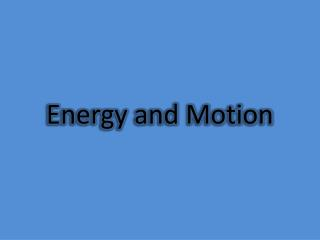DownloadDownload PresentationEnergy and Motion

# Energy and Motion

Télécharger la présentation## Energy and Motion

- - - - - - - - - - - - - - - - - - - - - - - - - - - E N D - - - - - - - - - - - - - - - - - - - - - - - - - - -
##### Presentation Transcript

1. Energy and Motion

2. Energy

3. Energy The ability to do work or cause change. • Two forms of energy: kinetic and potential • Kinetic Energy = Energy of Motion • Potential Energy = Stored energy, due to position or shape of the object.

4. Potential Energy • Potential to do work • Energy that can be transformed for later use • Elastic potential energy- potential energy associated with objects that can be stretched or compressed • Gravitational Potential Energy- potential energy that depends on the height of the object.

5. Forms of Energy Potential and Kinetic energy come in many forms: • Mechanical- P/K- motion or position, ex. Frog hopping • Thermal- P+K- total energy of particles • Chemical- P-stored in chemical bonds of chemicals, cells, a match to light a candle, etc.

6. Forms of Energy cont. • Electrical- K- moving electric charge batteries, lights, computers, etc. • Electromagnetic- K- energy that travels in waves- ex. Visible light • Nuclear- P- stored in the nucleus of an atom. Ex. Nuclear power.

7. Energy Transformations • Energy transformation or energy conversion- the change from one form of energy to another. • Most forms of energy can be converted into other forms. • Examples: • Mechanical of moving water to electrical energy (electricity) • Toaster- electrical to thermal (heat) • Motor- electrical energy to mechanical (moving parts of a machine) • Chemical energy in food to mechanical (muscle movement) thermal energy (heat) in your body

8. Energy Transformations • Typically several conversions are required to finish a task. • Examples of Potential to Kinetic Energy and Visa Versa: Juggling, Waterfall, Pole Vault, Pendulum, Sliding down a slide, a rock falling off a cliff etc. Question: What conversion takes place when an electric lamp is turned on?

9. Energy Transformations Problem 1: A student is investigating potential and kinetic energy by stretching a spring across a table. When the student lets go, the spring recoils. At which time is potential energy in the spring being converted into kinetic energy in this system? A. when the spring is stretching B. when the spring is fully stretched C. when the spring is recoiling D. when the spring is fully recoiled

10. Energy Transformations Problem 2:The diagram below shows some positions in the path of pendulum swinging from a fixed point called a fulcrum. The pendulum is raised to the start position and released. At which two numbered positions is the potential energy of the pendulum most likely the same? A. position 1 and position 3 B. position 1 and position 4 C. position 2 and position 3 D. position 2 and position 4

11. Motion

12. What is Motion? An object is in motion when its distance from another object is changing. An object is in motion if it changes position relative to a reference point. Change in Distance or Direction indicates motion

13. SPEED The rate of change in position, OR how fast something moves. CALCULATION: Speed = Distance Time Different kinds of Speed: Constant Speed, Average Speed and Instantaneous Speed.

14. What is the difference between SPEED and VELOCITY??? DIRECTION VELOCITY describes both SPEED and DIRECTION Velocity = Distance Time

15. EXAMPLE 1 What is the speed of a plane that traveled 3,500 miles from Boston to California in 7.0 hours? What is its velocity?

16. Changing the Equation for Speed Speed = Distance Time Distance = Speed x Time Time = Distance Speed

17. EXAMPLE 2 It took 4.5 hours for a train to travel the distance between two cities at a velocity of 110 miles/hr. How many miles lie between the two cities?

18. Graphing Speed Distance-Time Graphs What is the slope of A? What is the slope of B? How does C compare to A? What does D represent? Slope = Y2-Y1 X2-X1 The slope on a distance-time graph shows: SPEED D B C A